JEE  >  Test: MCQs (One or More Correct Option): Straight Lines and Pair of Straight Lines | JEE Advanced

# Test: MCQs (One or More Correct Option): Straight Lines and Pair of Straight Lines | JEE Advanced

Test Description

## 9 Questions MCQ Test Maths 35 Years JEE Main & Advanced Past year Papers | Test: MCQs (One or More Correct Option): Straight Lines and Pair of Straight Lines | JEE Advanced

Test: MCQs (One or More Correct Option): Straight Lines and Pair of Straight Lines | JEE Advanced for JEE 2023 is part of Maths 35 Years JEE Main & Advanced Past year Papers preparation. The Test: MCQs (One or More Correct Option): Straight Lines and Pair of Straight Lines | JEE Advanced questions and answers have been prepared according to the JEE exam syllabus.The Test: MCQs (One or More Correct Option): Straight Lines and Pair of Straight Lines | JEE Advanced MCQs are made for JEE 2023 Exam. Find important definitions, questions, notes, meanings, examples, exercises, MCQs and online tests for Test: MCQs (One or More Correct Option): Straight Lines and Pair of Straight Lines | JEE Advanced below.
 1 Crore+ students have signed up on EduRev. Have you?
*Multiple options can be correct
Test: MCQs (One or More Correct Option): Straight Lines and Pair of Straight Lines | JEE Advanced - Question 1

### Three lines px + qy + r = 0, qx + ry + p = 0 and rx + py + q = 0 are concurrent if (1985 - 2 Marks)

Detailed Solution for Test: MCQs (One or More Correct Option): Straight Lines and Pair of Straight Lines | JEE Advanced - Question 1

For concurrency of three lines px + qy + r = 0; qx + ry + p = 0; rx + py + q = 0 We must have,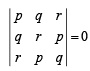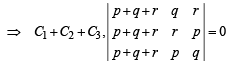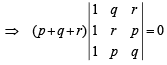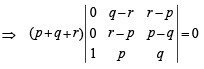⇒ (p + q + r) (pq – q2 – rp + rq – r2 + pr + pr – p2) = 0

⇒ (p + q + r) (p2 + q2 + r2 – pq – pr – rq ) = 0

⇒ p3 + q3 + r3 – 3pqr = 0

It is clear that a, b, c are correct options.

*Multiple options can be correct
Test: MCQs (One or More Correct Option): Straight Lines and Pair of Straight Lines | JEE Advanced - Question 2

### The points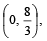(1, 3) and (82, 30) are vertices of

Detailed Solution for Test: MCQs (One or More Correct Option): Straight Lines and Pair of Straight Lines | JEE Advanced - Question 2

Let A (0, 8/3), B (1, 3) and C (82, 30).

Now, slope of line AB =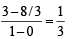Slope of line BC =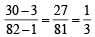⇒ AB || BC and B is common point.
⇒ A, B, C are collinear.

*Multiple options can be correct
Test: MCQs (One or More Correct Option): Straight Lines and Pair of Straight Lines | JEE Advanced - Question 3

### All points lying inside the triangle formed by the points (1, 3), (5, 0) and (–1, 2) satisfy (1986 - 2 Marks)

Detailed Solution for Test: MCQs (One or More Correct Option): Straight Lines and Pair of Straight Lines | JEE Advanced - Question 3

Substituting the co-ordinates of the points (1, 3), (5, 0) and (– 1, 2) in 3x + 2y, we obtain the value 8, 15 and 1 which are all +ve. Therefore, all the points lying inside the triangle formed by given points satisfy 3x + 2y ≥ 0.
Substituting the co-ordinates of the given points in 2x + y – 13, we find the values – 8, – 3 and – 13 which are all –ve.
So, (b) is not correct.
Again substituting the given points in 2x – 3y – 12 we get – 19, – 2, – 20 which are all –ve.
It follows that all points lying inside the triangle formed by given points satisfy 2x – 3y – 12 ≤ 0.
So, (c) is the correct answer.
Finally substituting the co-ordinates of the given points in – 2x + y, we get 1, – 10 and 4 which are not all +ve.
So, (d) is not correct.
Hence, (a) and (c) are the correct answers.

*Multiple options can be correct
Test: MCQs (One or More Correct Option): Straight Lines and Pair of Straight Lines | JEE Advanced - Question 4

A vector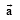has components 2p and 1 with respect to a rectangular cartesian system. This system is rotated through a certain angle about the origin in the counter clockwise sense. If, with respect to the new system , ar has components p + 1 and 1, then (1986 - 2 Marks)

Detailed Solution for Test: MCQs (One or More Correct Option): Straight Lines and Pair of Straight Lines | JEE Advanced - Question 4

Consider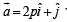with respect to original axes and a = ( p + 1) i+j with respect to new axes.
Now, as length of vector will remain the same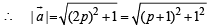⇒ p2 + 2p + 2 = 4p2 + 1

⇒ 3p2 – 2p – 1 = 0

⇒ p = 1 or – 1/3

∴ (b) is the correct answer.

*Multiple options can be correct
Test: MCQs (One or More Correct Option): Straight Lines and Pair of Straight Lines | JEE Advanced - Question 5

If (P(1, 2), Q(4, 6), R(5, 7) and S(a, b) are the vertices of a parallelogram PQRS, then (1998 - 2 Marks)

Detailed Solution for Test: MCQs (One or More Correct Option): Straight Lines and Pair of Straight Lines | JEE Advanced - Question 5

PQRS will represent a parallelogram if and only if the mid-point of PR is same as that of the mid-point of QS.
That is, if and only if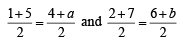⇒ a = 2 and b = 3.

*Multiple options can be correct
Test: MCQs (One or More Correct Option): Straight Lines and Pair of Straight Lines | JEE Advanced - Question 6

The diagonals of a parallelogram PQRS are along the lines x +3y = 4 and 6x – 2y = 7. Then PQRS must be a. (1998 - 2 Marks)

Detailed Solution for Test: MCQs (One or More Correct Option): Straight Lines and Pair of Straight Lines | JEE Advanced - Question 6

Slope of x + 3y = 4 is – 1/3 and slope of  6x – 2y = 7 is  3.
Therefore, these two lines are perpendicular which shows that both diagonals are perpendicular. Hence PQRS must be a rhombus.

*Multiple options can be correct
Test: MCQs (One or More Correct Option): Straight Lines and Pair of Straight Lines | JEE Advanced - Question 7

If the vertices P, Q, R of a triangle PQR are rational points, which of the following points of the triangle PQR is (are) always rational point(s)? (1998 - 2 Marks)

Detailed Solution for Test: MCQs (One or More Correct Option): Straight Lines and Pair of Straight Lines | JEE Advanced - Question 7

Since the co-ordinates of in the centre depend on lengths of side of Δ.

∴ it  can have irrational coordinates

*Multiple options can be correct
Test: MCQs (One or More Correct Option): Straight Lines and Pair of Straight Lines | JEE Advanced - Question 8

Let L1 be a strainght line passing through the origin and L2 be the straight line x +y = 1. If the intercepts made by the circle x2 + y2 - x + 3y= 0 on L1 and L2 are equal, then which of the following equations can represent L1? (1999 - 3 Marks)

Detailed Solution for Test: MCQs (One or More Correct Option): Straight Lines and Pair of Straight Lines | JEE Advanced - Question 8

We know that length of intercept made by a circle on a line is given by =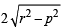where p = ⊥ distance of line from the centre of the circle.

Here circle is x2 + y2 – x + 3y = 0 with centre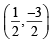and radius =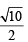L1 : y = mx (any line through origin)

L2 : x + y – 1 = 0 (given line)

ATQ circle makes equal intercepts on L1 and L2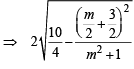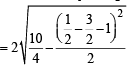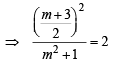⇒ m2 + 6m + 9 = 8m2 + 8   ⇒ 7m2 – 6m – 1 = 0

⇒ 7m2 – 7m + m – 1 = 0  ⇒ (7m + 1) (m – 1) = 0

⇒ m = 1, – 1/7

∴ The required line L1 is y = x  or y =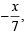i.e., x – y = 0 or x + 7y = 0.

Test: MCQs (One or More Correct Option): Straight Lines and Pair of Straight Lines | JEE Advanced - Question 9

For a > b > c > 0, the distance between (1, 1) and the point of intersection of the lines ax + by + c = 0 and bx + ay + c = 0 is less than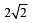. Then (JEE Adv. 2013)

Detailed Solution for Test: MCQs (One or More Correct Option): Straight Lines and Pair of Straight Lines | JEE Advanced - Question 9

Solution :- ax+by+c=0 ;equation(i)

bx+ay+c=0 ;equation(ii)

equation(i)×a−equation(ii)×b

a2x+aby+ac−b2x−aby+bc=0

⇒x(a2−b2)+c(a−b)=0

⇒x(a−b)(a+b)+c(a−b)=0

Dividing both sides by (a−b), we get,

x(a+b)+c=0

⇒x = −c/(a+b)

Similarly equation(ii)×a−equation(i)×b, yields

y = −c/(a+b)

⇒(x,y)=(−c/(a+b), − c/(a+b))

Given that distance between (x,y) and (1,1) is less than 2√2

√{1−(−c/(a+b))}2 + {1−(−c/(a+b))}2

√((a+b+c)/a+b)2 + ((a+b+c)/a+b)2  < 2√2

= √2((a+b+c)/a+b)2 < 2√2

Squaring both sides, we get,

2((a+b+c)/a+b)2<8

⇒((a+b+c)/a+b)2<4

⇒Let a+b=t

⇒ [(t+c)2]/t2<4

⇒(t+c)2<4t2

⇒4t2−(t+c)2>0

⇒4t2−t2−c2−2tc>0

⇒3t2−2tc−c2>0

⇒3t2−3tc+tc−c2 >0

⇒3t(t−c)+c(t−c)>0

⇒(3t+c)(t−c)>0

As t=a+b

⇒(3a+3b+c)(a+b−c)>0

Given that a>b>c>0

⇒3a+3b+c>0

Hence for product of (3a+3b+c) and (a+b−c) to be positive, (a+b−c) should also be

positive.

⇒a+b−c>0

## Maths 35 Years JEE Main & Advanced Past year Papers

132 docs|70 tests
 Use Code STAYHOME200 and get INR 200 additional OFF Use Coupon Code
Information about Test: MCQs (One or More Correct Option): Straight Lines and Pair of Straight Lines | JEE Advanced Page
In this test you can find the Exam questions for Test: MCQs (One or More Correct Option): Straight Lines and Pair of Straight Lines | JEE Advanced solved & explained in the simplest way possible. Besides giving Questions and answers for Test: MCQs (One or More Correct Option): Straight Lines and Pair of Straight Lines | JEE Advanced, EduRev gives you an ample number of Online tests for practice

## Maths 35 Years JEE Main & Advanced Past year Papers

132 docs|70 tests